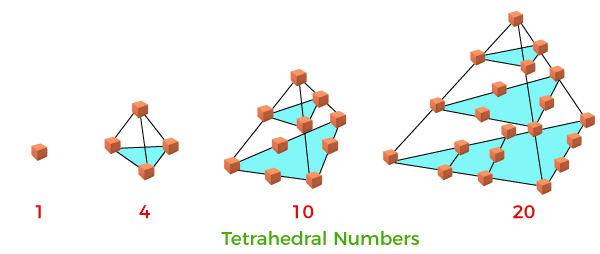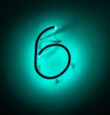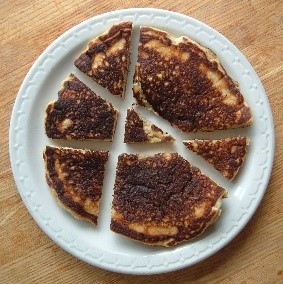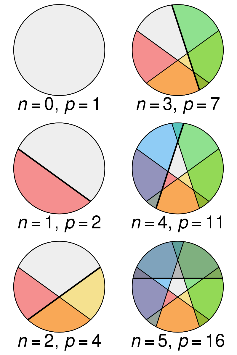# Interesting Numbers To Show Your Class

In this blog we cover five interesting sequences of numbers to show your class. They are outside the scope of the curriculum, and will be a great way to ignite the curiosity of your pupils.

There is a brief introduction to each sequence, and hopefully this article will be a springboard for further investigation, by teachers and pupils alike.

### Tetrahedral NumbersImage Credit: JavaTpoint - Tetrahedral Number in Java

Your pupils might be able to remember what a triangular number is. Tetrahedral Numbers are made by adding up their more famous cousins, the triangular numbers. (Triangular Numbers are: 1,3,6,10,…)

To work out the fourth Tetrahedral Number, add up the first four Triangular Numbers.

The 4th Tetrahedral Number 1+3+6+10=20.

As you can see from the picture above, tetrahedral numbers look like tetrahedrons when they are arranged in the right way. Tetrahedral numbers have real world applications in Sphere Packing, which is the science of packing spheres (often applied to molecules) in the most efficient way possible.

### Perfect NumbersImage Credit: Photo by Maximalfocus on Unsplash

A Perfect Number is equal to the sum of its proper factors. Proper factors mean that you aren’t including the number itself.

6 is the smallest Perfect Number: 6=1+2+3

Question to ask: Can your pupils find the next Perfect Number less than 30?

Below is a list of the first 10 Perfect Numbers. The 10th Perfect Number is absolutely huge. It is in fact an unsolved problem in mathematics as to whether there are infinitely many perfect numbers. It is really good for pupils to find out that mathematicians don’t know everything.

First ten Perfect Numbers: 6, 28, 496, 8128, 33550336, 8589869056, 137438691328, 2305843008139952128, 2658455991569831744654692615953842176, 191561942608236107294793378084303638130997321548169216

Perfect Numbers don’t however just appear randomly, there is a fascinating connection between Perfect numbers and Mersenne Primes as can be seen in Numberphile's Perfect Numbers and Mersenne Primes video. It only gets better – All Perfect Numbers are themselves Triangular Numbers as well!

Related to the concept of Perfect Numbers, are the ideas of Abundancy and Deficiency. As you might be able to guess, If the proper factors add up to less than the number, it is deficient. If they add up to more than the number, it is abundant. Choose some (reasonably sized) numbers for your pupils to classify as abundant, deficient or perfect.

### Lazy Caterer’s Sequence

Imagine you have a round pizza and you are going to make straight cuts at random. What is the maximum number of pieces you could divide your pizza up into? For one cut it is two pieces. For two cuts, it is also easy, it is four pieces. For three cuts it is a bit harder, but with some work we can see it is seven pieces.Image Credit: Wikipedia - Lazy caterer's sequence

With three cuts the maximum number of pieces is seven.

We are starting to form a sequence: 1,2,4,7,… It starts with the number 1, because we want to show what will happen with zero cuts. Ask your pupils to try and generate the next number in the sequence.Image Credit: Wikipedia - Lazy caterer's sequence

The Lazy Caterer’s Sequence [1,2,4,7,11,16,…] is a very interesting sequence. It has a quadratic nth term, and the sequence can also be found inside Pascal’s Triangle. (Can you spot it?)

Keith Numbers

Keith Numbers are relatively young, having only been ‘discovered’ by Mike Keith in 1987. They are part of what is called Recreational Mathematics, which is the art of doing mathematics just for fun.

How to decide if your number is a Keith Number:

Let’s test the number 197

Step 1. Decide how many digits your number has. For 197, n=3, where n is the number of digits.

Step 2. Add up the digits of your number. 1+9+7 = 17

Step 3. Add up the last n=3 numbers in the sum above. 9+7+17 = 33

Step 4. Repeat this process to see if your original number appears. (If the original number never appears it is not a Keith Number.)

7+17+33 = 57

17+33+57= 107

33+57+107 = 197 [Hooray, we found 197, the original number – 197 is a Keith Number!!!]

How it works for a two-digit number:

Let’s test the number 19

Step 1. Decide how many digits your number has. For 19, n=2, where n is the number of digits.

Step 2. Add up the digits of your number. 1+9 = 10

Step 3. Add up the last n=2 numbers in the sum above. 9+10 = 19

[We found 19, the original number – 19 is a Keith Number!!!]

There is no known purpose to Keith Numbers apart from the sheer mathematical pleasure that they give.  History has however proved time and time again that recreational mathematics can lead to unexpected breakthroughs further down the line.

Check out the Numberphile Video on Keith Numbers.

### Look and Say Sequence

The following sequence is one of the most bizarre that you will ever meet. If you have not encountered it before, take a few minutes to see if you can work out what the pattern is.

1, 11, 21, 1211, 111221, 312211, 13112221, 1113213211, …

What you need to do is to read it out loud – i.e. ‘Look and Say’ what you see.

The sequence starts with the number 1.

Say “One one.” Write down 11.

Say “Two ones.” Write down 21

Say “One two, one one.” Write down 1211

Say “One one, one two, two ones.” Write down 111221

And so on…..

Is the Look and Say Sequence Mathematical Nonsense?

This sequence may look like mathematical nonsense, but your pupils will enjoy generating the next few terms of the sequence and some of them might even like to take their sequence home as a party trick to show their families.

When this sequence was shown to John Horton Conway, one of the greatest mathematicians of the 20th Century, he couldn’t resist getting his teeth stuck into it, and went on to show that it has some fascinating mathematical properties.

Amongst other things, John Conway started taking two consecutive terms and working out the ratio between how many digits they have.

For example, take the 7th and 8th terms: 13112221 (has 8 digits), 1113213211 (has 10 digits). John Conway was looking at the ratio: 10/8 = 1.25.  If you keep doing this for consecutive pairs of numbers, as they get really large, the ratio will tend towards 1.303577… which is now known as Conway’s Constant. This sequence proves that mathematics really is everywhere.

John Horton Conway talks about Look-and-Say Numbers on a Numberphile video.

One idea is for pupils to make up their own sequence of numbers and to give it a name. What do they come up with and can they spot any patterns?